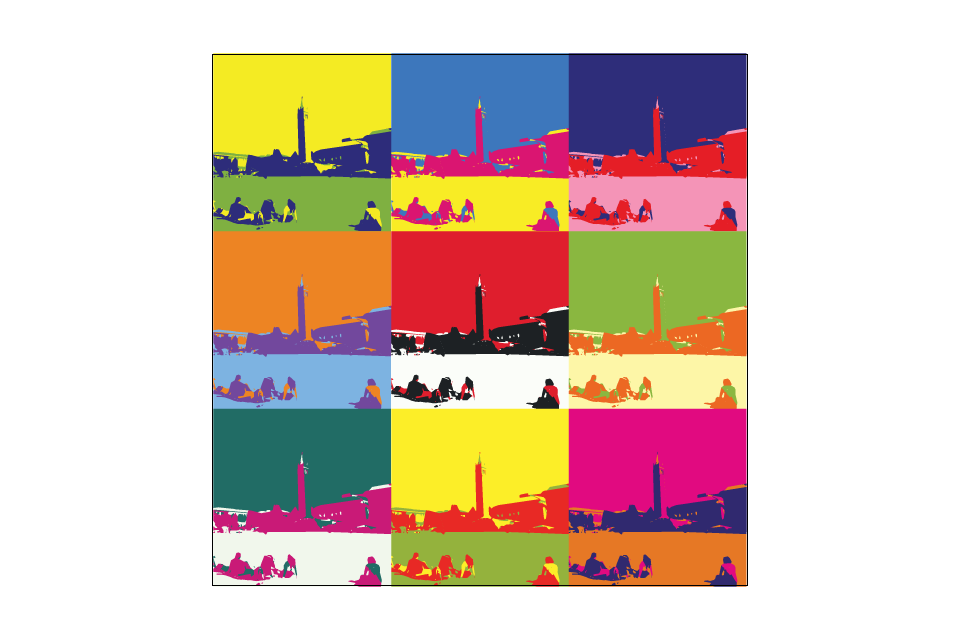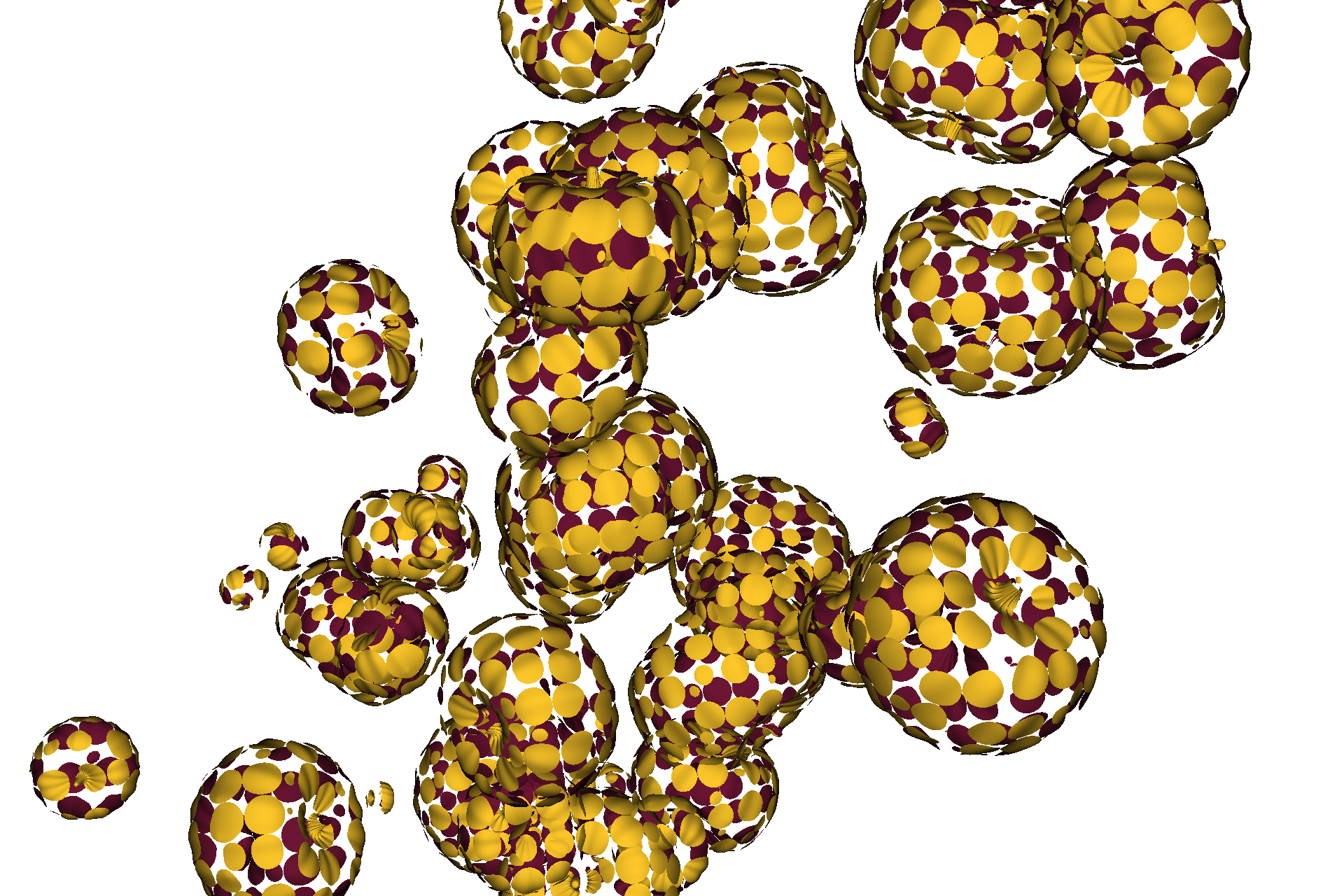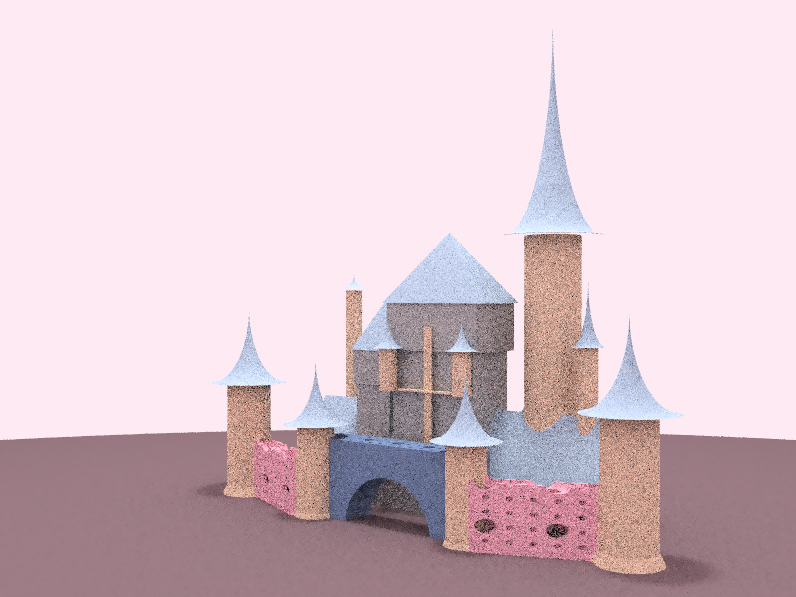Discussion 1

Bullets

• Rasterization
• Point in triangle test (three-line test)
• Edge cases
• Artifacts
• Jaggies
• Antialiased rasterization
• Spatial domain
• Frequency domain
• Filtering & Convolution
• Interactive demo
• Nyquist Frequency
Half of the sampling frequency
• Super sampling

A little bit about me

• Took 184 this time last year
• Did some interesting artworks in the competition with project partnersCampanile in an Andy Warhol stylePumpkins in a Yayoi Kusama styleCastle (wishing to be in a Disney style)Partnered with Diyu Luo & Jiejun Luo

Graphics pipeline

• Per-vertex ops (may include texturing)
• Rasterization (focus for today)
• Per-fragment ops (may include texturing)
• Framebuffer ops

Real world example

Pixel data

• Often times each channel stored on disk as unsigned char, 8 bits
Range from 0 (0x00) to 255 (0xff) (inclusive)

• Often computed in float, 32 bits
Using range from 0 to 1 (inclusive)
Or in half, 16 bits, if not needing much precision

Pixel order

Stored in a continuous array, the pixels are in row-major order (first, second, third row…)

What'll be the index for a pixel at (x, y) = (4, 5) for an image of size width * height?

5 * width + 4

Point in triangle test $R^2$

Point in triangle test demo

Nyquist theorem

Nyquist frequency: Half the sampling frequency
We get no aliasing from frequencies less than the Nyquist frequency.

As an example, for an image of stripes (black & white), if we're sampling at every 16px, stripes at cycle every 32px or more will result in no aliasing.

Also, if there are stripes that cycle at every 8px (4px black, then 4px white), we'd want to sample at every 4px to avoid aliasing.

Filtering

Convolution in the spatial domain equals to point-wise multiplication in the frequency domain.

Image fourier analysis demo

Convolution example

GitHub repo to follow along: https://github.com/cal-cs184/disc01

CS 184 C++ Primer: https://cs184.eecs.berkeley.edu/sp19/article/11/c-basics

Useful ways to iterate over a std::vector<unsigned char> named vec:

for (unsigned long i = 0; i < vec.size(); i++) {
// Get with vec[i]
// Set with vec[i] = ...
}
for (unsigned char c : vec) {
// Get with c
}
for (unsigned char &c : vec) {
// Get with c
// Set with c = ...
}

Useful functions from lodepng:

/*
Converts PNG file from disk to raw pixel data in memory.
Same as the other decode functions, but instead takes a filename as input.
*/
unsigned decode(std::vector<unsigned char>& out, unsigned& w, unsigned& h,
const std::string& filename,
LodePNGColorType colortype = LCT_RGBA, unsigned bitdepth = 8);
/*
Converts 32-bit RGBA raw pixel data into a PNG file on disk.
Same as the other encode functions, but instead takes a filename as output.
NOTE: This overwrites existing files without warning!
*/
unsigned encode(const std::string& filename,
const std::vector<unsigned char>& in, unsigned w, unsigned h,
LodePNGColorType colortype = LCT_RGBA, unsigned bitdepth = 8);

Annotated reference solution

void Filter::read(std::string filename) {

// Open the rile
std::ifstream file(filename);

// Read width & height
file >> width >> height;

// Resize the kernel
unsigned int size = width * height;
kernel.resize(size);

// Read the kernel data
for (int i = 0; i < size; i++) {
file >> kernel[i];
}

// Normalize the filter
normalize();

}

void Filter::normalize() {

// Calc the sum
float sum = 0;
for (float k : kernel) {
sum += k;
}

// Adjust the kernel data
for (float &k : kernel) {
k /= sum;
}

}

const float& Filter::at(int x, int y) const {

return kernel[y * width + x];

}

void Image::read(std::string filename) {

// Clear the image
data.clear();

// Load the image with lodepng
lodepng::decode(data, width, height, filename);

}

void Image::write(std::string filename) const {

// Write the image with lodepng
lodepng::encode(filename, data, width, height);

}

uint8_t* Image::at(int x, int y) {

// Each pixel takes 4 values
return &(data[4 * (y * width + x)]);

}

Image Image::operator*(const Filter& filter) {

// New output image
Image oimage(width, height);

// Iterate every pixel on the output image
for (int oy = 0; oy < height; oy++) {
for (int ox = 0; ox < width; ox++) {

// Temporary accumulator
float sum = {0, 0, 0, 0};

// Iterate over the filter
for (int fy = 0; fy < filter.height; fy++) {
for (int fx = 0; fx < filter.width; fx++) {

int ix = ox - filter.width / 2 + fx;
int iy = oy - filter.height / 2 + fy;

// Discard pixels outside the image bounds
if (ix < 0 || iy < 0 || ix >= width || iy >= height) continue;

float f = filter.at(fx, fy);

sum += at(ix, iy) * f;
sum += at(ix, iy) * f;
sum += at(ix, iy) * f;
sum += at(ix, iy) * f;

}
}

// Output pixel to write to
uint8_t *opixel = oimage.at(ox, oy);

opixel = sum;
opixel = sum;
opixel = sum;
opixel = sum;

}
}

// Return the image
return oimage;

}